Home Introduction Assignments References Acknowledgments

Angela B. Shiflet
Wofford College
shifletab@wofford.edu
http://www.wofford.edu/ecs/

## Abstract

"Spreading of Fire" provides twelve assignments on cellular automaton simulation that, depending on the project, are appropriate for CS1, CS2, computer graphics, or modeling and simulation. Each of the assignments involves simulating the spread of fire in order to gain insight into the situation. Many investigate the impact of lightning or of a metric indicating fire conditions, such as atmospheric humidity and wood moisture. For example a plot of the simulated data in one assignment is a logistic (elongated S) curve, illustrating how volatile the spreading of fire situation is when the probability of a tree catching on fire from a neighbor is around 50%. With such a probability, which indicates fire conditions, such as humidity, the fire is likely to die out quickly or spread rampantly.

The "Spreading of Fire" Nifty Assignment website explains the cellular automaton fundamentals so that students with only a basic knowledge of two-dimensional arrays and random number generation can develop their own simulations. Moreover, for some of the assignments, students can use a graphics library to "bring to life" their results with computer animation. Such real-world type applications, some with visualizations, make great team assignments that are appealing, instructive, and fun for the students.

Meta-information

 Summary Spreading of Fire—Variety of assignments on cellular automaton simulation of the spreading of fire Topics Two-dimensional arrays; simulation techniques; for some assignments, visualizations Audience Depending on the assignment, CS1, CS2, computer graphics, modeling and simulations Difficulty Moderate Strengths Provides real-world type applications; introduces the concepts of cellular automaton simulation; presents good team assignments.  A plot of the data in Assignment 3 is a logistic (elongated S) curve, illustrating how volatile the spreading of fire situation is when the probability of a tree catching on fire from a neighbor is around 50%.  With such a probability, which indicates fire conditions, such as humidity, the fire is likely to die out quickly or spread rampantly. Weaknesses Students must understand concepts associated with cellular automata and then map those into programming concepts, although the mapping is straightforward.  Checking program output for correctness is more difficult because of the stochastic nature of a simulation, but checking code for correctness is straightforward. Dependencies Students must know how to use two-dimensional arrays and ordered sequences (lists), as well as how to use a pseudo random number generator.  If the assignment employs visualization, students must be able to use a graphics library. Variants Twelve possible assignments are listed.  Since 2001, we have implemented various versions using Mathematica in the course Modeling and Simulation for the Sciences, which has no prerequisites.  Simulation and visualizations have also been implemented in Python, Maple, and MATLAB.

Detailed Description

The assignment write-up is below, here in pdf format, and here in doc format.

Fighting fires in Southern California or anywhere else is a very risky job, where loss of life is a real possibility.  Proper training is essential.  In the United States, the National Fire Academy, established in 1974, presents courses and programs that are intended “to enhance the ability of fire and emergency services and allied professionals to deal more effectively with fire and related emergencies” (Studebaker 2003).  The Academy has partnered with private contractors and the U.S. Forest Service to develop a three-dimensional land fire fighting training simulator.  This simulator exposes trainees to a convincing fire propagation model, where instructors can vary fuel types, environmental conditions, and topography. Responding to these variables, trainees may call for appropriate resources and construct fire lines.  Instructors may continue to alter the parameters, changing fire behavior.  Students can review the results of their decisions, where they can learn from their mistakes in the safety of a computer laboratory (Studebaker 2003).

Modeling is the application of methods to analyze complex, real-world problems in order to make predictions about what might happen with various actions.  When it is too difficult, time-consuming, costly, or dangerous to perform experiments, the modeler might employ computer simulation, or having a computer program imitate reality, in order to study situations and make decisions. By simulating a process, he or she can consider various scenarios and test the effect of each.

The Problem

Each of the assignments below involves simulating the spread of fire in order to gain insight into the situation.  Many investigate the impact of lightning or of a metric, or numeric measure, indicating fire conditions, such as humidity of the atmosphere and dryness of the wood.

Cellular Automaton Simulation

One way to look at the world is to study a process is as a group of smaller pieces (or cells or sites) that are somehow related.  Each piece corresponds to an area (or volume) in the world.  Each piece can be associated with one of several possible states at any given time. One convenient way to lay out the world is as a rectangular grid of cells.  Rules specify how a cell changes state over time based on the states of the cells around it.

A computer simulation involving such a system is a cellular automaton.  Cellular automata are dynamic computational models that are discrete in space, state, and time.  We picture space as a one-, two-, or three-dimensional  (also sometimes called an array or lattice).  A site (or cell), of the grid has a state, and the number of states is finite.  Rules (or transition rules) specifying local relationships and indicating how cells are to change state, regulate the behavior of the system.  An advantage of such grid-based models is that we can visualize through informative animations the progress of events.  For example, we can view a simulation of the movement of ants toward a food source, the propagation of infectious diseases, heat diffusion, distribution of pollution, or the motion of gas molecules in a container.  The current assignment develops a two-dimensional computer simulation for the spread of fire.

Much of this material and these assignments are derived from Introduction to Computational Science:  Modeling and Simulation for the Sciences (http://wofford-ecs.org/IntroComputationalScience/index.htm) by Angela Shiflet and George Shiflet (Shiflet, 2006).  The original inspiration for this assignment was the module "Fire!!" and associated cellular automaton simulation from The Shodor Education Foundation (http://www.shodor.org) (Shodor, fire).  Run the Shodor "Fire!!" applet at URL http://www.shodor.org/interactivate/activities/fire1/index.html at the Shodor Foundation or watch a movie of a simulation run hereFigure 4 below displays several frames in the visualization of a simulation.  This assignment is a simplification of the Shodor simulation in that a tree burns down in one time step and a cell with a burned tree has the same state as an empty cell.

## Initializing the System

In many simulations, we model a dynamic area under consideration with an n-by-n grid (or lattice or a two-dimensional square array or matrix) of numbers, where a number represents a state (see Figure 1).  Each cell in the grid contains a value representing a characteristic of a corresponding location.  For example, in a simulation for the spread of fire, a cell can contain one of three values:  0 to indicate an empty cell with no tree or a burnt tree, 1 to indicate a cell with a non-burning tree, or 2 to indicate a cell with a burning tree.  Table 1 lists these values and meanings along with associated names EMPTY, TREE, and BURNING that have values of 0, 1, and 2, respectively.  We initialize these constants at beginning and employ the descriptive names throughout the program.  Thus, the code is easier to understand and to change.

Figure 1    Cells to model area with associated numeric values

a.

b.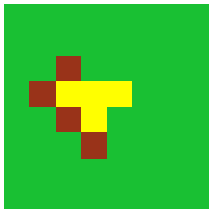1 1 1 1 1 1 1 1 1 1 1 1 1 1 1 1 1 1 2 1 1 1 1 1 1 2 0 0 0 1 1 1 1 1 2 0 1 1 1 1 1 1 1 2 1 1 1 1 1 1 1 1 1 1 1 1 1 1 1 1 1 1 1 1

Table 1      Cell values with associated constants and their meanings

 Value Constant Meaning 0 EMPTY The cell is empty ground or a burnt tree containing no tree. 1 TREE The cell contains a tree that is not burning. 2 BURNING The cell contains a tree that is burning.

How do we address the problem of the edge of the world at interest?  For this simulation, we assume our world is surrounded by empty cells—that is, cells that contain no trees or anything else that can burn.  The boundary of empty cells is similar to a firebreak or an area with no trees; proximity to such a boundary cell cannot cause an internal tree to catch fire.  This insulating boundary is called an absorbing boundary conditionFigure 2 depicts an initial discrete stochastic system with green indicating a non-burning tree, red a burning tree, and yellow an empty cell.

Figure 2    Grid of trees with one burning tree in the middle and with each cell on an extended boundary having a value of EMPTY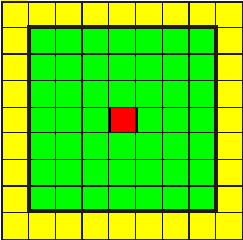## Updating Rules

How do we model the spread of fire?  If a small area of a forest catches fire, then that fire spreads to a neighboring cell with some probability determined by factors such as wind, precipitation falling, or dryness of the leaves and timber.  We model transition rules by a function spread.  At each simulation iteration, we apply spread to each cell site to determine its value—EMPTY, TREE, or BURNING—at the next time step.  A cell's value at the next instant depends on the cell's current value (site) and the values of its neighbors to the north (N), east (E), south (S), and west (W).  Thus, we use five numeric parameters—site, N, E, S, and W—for spread.  In a call to this function, each argument is one of the values EMPTY, TREE, or BURNINGFigure 3 pictures the cells that determine a site's next value along with their indices, where the indices for site are (i, j).  To simplify programming for this simulation, we assume the state of a diagonal cell to the northeast, southeast, southwest, or northwest does not have an impact on a site's value at the next iteration.  Thus, the term neighbor refers to one of the cells directly to the north, east, south, and west of a site's cell.

Figure 3    Cells that determine a site's next value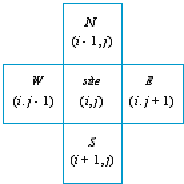Updating rules apply to different situations:  If a site is empty (cell value EMPTY), it remains empty at the next time step.  If a tree grows at a site (cell value TREE), at the next instant the tree may or may not catch fire (value BURNING or TREE, respectively) due to fire at a neighboring site.  We make the simplifying assumption that a burning tree (cell value BURNING) always burns down in one time step, leaving an empty site (value EMPTY) for the next time step.  We also assume that a site with empty ground and a site with a burnt tree are in the same state and have the value EMPTY.  We consider each situation separately.

When a tree is burning, the first argument, which is the site's value, is BURNING.  Regardless of its neighbors' situations, the tree burns down, so that at the next iteration of the simulation the site's value becomes EMPTY.  Thus, the relevant rule for the spread function has a first argument of BURNING; each of the other four arguments are immaterial; and the function returns value of EMPTY.

To continue development of this dynamic, discrete stochastic system, we employ the following additional probability:

probCatch – The probability of a tree in a cell catching fire if a tree in a neighboring cell is on fire.

Thus, if a site contains a tree (site value of TREE) and fire threatens the tree, probCatch is the probability that the tree will catch fire at the next time step.

To illustrate how a probability can be determined during a simulation run, suppose probCatch is 0.15 = 15%.  For each cell with the value TREE that has a burning neighbor, we generate a uniformly distributed random floating point number from 0.0 up to 1.0.  On the average, 15% of the time this random number is less than 0.15, while 85% of the time the number is greater than or equal to 0.15.

Thus, we employ the following logic:

if site is TREE and (N, E, S, or W is BURNING)

if a random number between 0.0 and 1.0 is less than probCatch

return BURNING

else

return TREE

Even if a tree has the potential to burn because of a neighboring burning tree, it may not because of conditions, such as dry weather.  Such a tree has a probability of probCatch of burning.

## Applying Spread to Each Cell

To run a simulation, we have to represent the passing of time and the state of the world at each time.  Time is represented in discrete units, such as one minute, one hour, one day, and so on.  At each discrete time step, the simulation program determines the state of each cell at the next time step based on the state of each cell at the current time.  Thus, the program runs a simulation by counting time steps and at each one producing a new grid for the next time step.  To that end, we define a function applySpread that takes a grid (mat) and a probability of the fire spreading to a cell (probCatch) and returns another grid with spread applied to each internal cell of mat.  The function applySpread applies spread(site, N, E, S, W) to each internal cell in grid mat—that is, applySpread does not process the cells on the boundary.  Thus, for i going through the indices for each internal row (not including the first and last rows) and for j going through the indices for each internal column (not including the first and last columns), applySpread obtains a cell value for a new (n + 2)-by-(n + 2) grid as the application of spread to each site with coordinates i and j and its neighbors with corresponding coordinates as in Figure 3.

## Simulation Program

To perform the simulation of spreading fire, we define a function fire with parameters for n, the internal grid size, or number of internal grid rows or columns; and probCatch, the probability of catching fire.  A number of simulation assignments appear below in the section Assignments.  In one version of the program, the simulation continues until no cell is on fire and returns a list of the initial and all subsequent grids. Pseudocode for this version of fire is as follows:

fire(n, probCatch)
Function to return a list of grids in a simulation of the spread of fire in a forest, where a cell value of EMPTY indicates  the    cell is empty; TREE, the cell contains a non-burning tree; and BURNING, a burning tree
Pre:

n is the size (number of rows or columns) of the internal grid and is positive.
probCatch is the probability (a number between 0.0 and 1.0) of a tree catching fire.
spread is the function for the updating rules of each cell.
Post:
A list of the initial grid and the grid at each time step of the simulation was returned.
Algorithm:

initialize forest to be an (n + 2)-by-(n + 2) grid of values, as follows:  EMPTY (no tree) cells are on the boundary and
TREE (non-burning tree) cells are in the interior except for one BURNING (burning tree) cell in the middle.

grids is assigned a list containing forest
do as long as a burning tree is in forest:
forest is assigned the result of call to applySpread with arguments forest and probCatch, which returns
an
(n + 2)-by-  (n + 2) grid with spread applied to each internal cell of  forest

grids is assigned the list with forest appended onto the end of grids

return grids

## Display Simulation

Visualization helps modelers understand the meaning of the simulation.  For each grid in the list returned by fire, we generate a graphic for a rectangular grid with yellow representing an empty site; green, a tree; and red, a burning tree.  In some assignments below, a function showGraphs with parameter graphList containing the list of grids from the simulation produces these figures.  We animate the sequence of graphics to view the changing forest scene.  Figure 4 displays several frames (time steps 0-5, 20-22, and 70-72) of a fire with n = 25 and probCatch = 0.55.

Figure 4    Several frames in an animation of the spreading of fire

 Initial grid Grid at time step 1 Grid at time step 2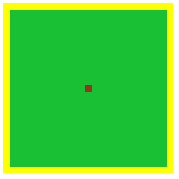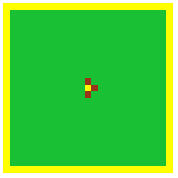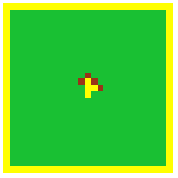Grid at time step 3 Grid at time step 4 Grid at time step 5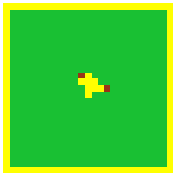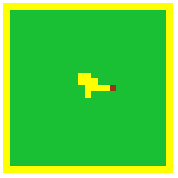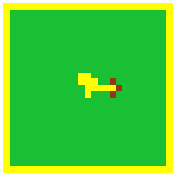Grid at time step 20 Grid at time step 21 Grid at time step 22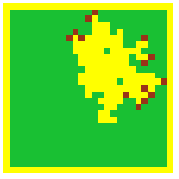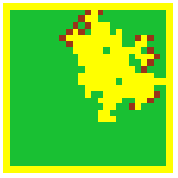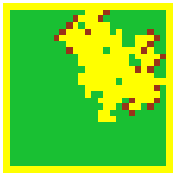Grid at time step 70 Grid at time step 71 Grid at time step 72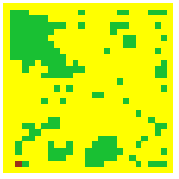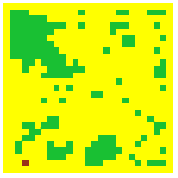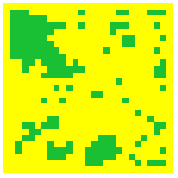1.      (This assignment does not require visualization.)  Develop a simulation for fire as described above.  Display the resulting grids by showing the value in each cell.  Run the program several times for each of the following situations.  Notice how the probability affects the spread of fire.
a.      probCatch is 0.2
b.      probCatch is 0.8
c.      probCatch is 0.5

2.      (This assignment is appropriate if the programming language provides access to graphics and animation libraries.)  Develop a simulation and animated visualization for fire as described above.  Run the program several times for each of the following situations and discuss the results.
a.      probCatch is 0.2
b.      probCatch is 0.8
c.      probCatch is 0.5

3.      (This assignment does not require visualization of the grids.)  Develop a fire simulation in which every cell in a 17-by-17 grid has a tree and only the middle cell's tree is on fire initially.  The simulation should have a parameter for probCatch, which is the probability of a tree adjacent to a burning tree catches fire.  A function should return the percent of the forest burned.  The program should run ten experiments with probCatch = 0%, 10%, 20%, 30%, …, and 100% and should run each simulation 10 times.  Also, have the code determine the average percent burned for each probability.  Plot the data for the 10 averages versus the percentages in one of the following ways:

• Plot the data by hand on paper
• Display the results, paste these data into a spreadsheet, and use the spreadsheet to create a graph
• Save the results to a file, read the file into a spreadsheet, and use the spreadsheet to create a graph
• Create a plot using some other appropriate software application

Notice how the probability affects the spread of fire (Shodor, fire).

4.      Do Assignment 1 or 2 using an initial random grid.  To initialize this discrete stochastic system, employ the following two probabilities:

probTree – The probability that a tree (burning or not burning) initially occupies a site.  Thus, probTree is the initial tree density measured as a percentage.

probBurning - If a site has a tree, the probability that the tree is initially burning, or that the grid site is BURNING.  Thus, probBurning is the fraction of the trees that are burning when the simulation begins.

Using the probabilities and cell values above, employ the following logic to initialize each cell in the grid for the forest:

if a random number is less than probTree                         // tree at site
if another random number is less than probBuring   // tree is burning
assign BURNING to the cell
else                                                                                    // tree is not burning
assign TREE to the cell
else                                                                                             // no tree at site
assign EMPTY to the cell

5.      Do Assignment 1, 2 or 4 considering that a tree might also catch fire because of a lightning strike.  Thus, when a tree is at a location (site value of TREE), at the next iteration the tree might be burning due to one of two causes, a burning tree at a neighboring site or a lightning strike at the site itself.  Let probLightning be the probability that the tree is struck by lightning.  With a probability of probCatch the tree will burn if struck by lightning.  For the tree to catch fire due to lightning, it must be hit and must catch fire.  Thus, lightning causes a tree to catch fire with the probability that is the product probLightning * probCatch.  For example, if a 0.2 = 20% chance exists for a lightning strike (probLightning) at the site of a tree and the probability of catching fire (probCatch) is 0.6 = 60%, then the tree burns with a probability of (0.2)(0.6) = 0.12 = 12%.  Two things must happen:  Lightning must hit, and the tree must catch fire from the strike.

In each of Assignments 6-8, revise the fire simulation in Assignment 1, 2, 4, or 5 to incorporate the indicated change.  Discuss the results.

6.      A tree takes two time steps to burn completely.

7.      A tree catches fire from neighboring trees with a probability proportional to the number of neighbors on fire.

8.      A tree grows instantaneously in a previously empty cell with a probability of probGrow.

9.      (This assignment is appropriate if the programming language provides access to graphics and animation libraries.)  Suppose a grid g has values for a forest grid, where a cell can be empty (value EMPTY = 0), a tree with the value (1 through 4) indicating the level of maturity from young to old, or a burning tree with the value indicating the intensity of the fire (5 for less intense or 6 for intense).  Develop a fire simulation with animation that shows a graphic representing g with yellow for an empty cell, a different level of green from pale to full green representing the age of a tree, light red for a less intense fire and full red for an intense fire.  Use constants, such as EMPTY, for the cell values.

10.    a.      Develop a fire simulation that considers wind direction and speed.  Have an accompanying animation.  Do not consider the possibility of lightning.  The simulation should have parameters for the probability (probTree) of a grid site being occupied by a tree initially, the probability of catching fire, the fire direction (value N, E, S, or W), wind level (value NONE = 0, LOW = 1, or HIGH = 2), coordinates of a cell that is on fire, and the number of cells along one side of the square forest.  The function should return the percent of the forest burned (Shodor, Better Fire).
b.      With a wind level of LOW (1) and a fixed probTree, vary wind direction and through animations observe the affects on the forest burn.  Discuss the results.
c.      Develop a program to run three experiments with wind levels of NONE = 0, LOW = 1, and HIGH = 2.  Have fixed wind direction and probTree. The program should conduct each experiment 10 times. Also, have the code determine the average percent burned for each level. Discuss the results.
d.      Develop a program to run eight experiments with no wind and probTree = 10%, 20%, 30%, …, and 90%.  The program should conduct each experiment ten times. Also, have the code determine the average percent burned for each probability.  Plot the data and fit a curve to the data.  Discuss the results.

11.    Develop a fire simulation in which a tree once hit by lightning in one time step takes five additional time steps to burn.  (See Assignment 5.)  The fire can spread from the burning tree to a neighboring tree with a certain probability only on the second, third, and fourth time steps after the lightning strike.

12.    Develop a fire simulation with accompanying animation in which a section of the forest is damper and, hence, harder to burn.